Hello, Guest!
 Instructional Focus DocumentPrecalculus
 TITLE : Unit 10: Vectors SUGGESTED DURATION : 8 days

#### Unit Overview

Introduction
This unit bundles student expectations that address representing, using, and applying vectors and vector operations, including vector addition and multiplication of a vector by a scalar. Concepts are incorporated into both mathematical and real-world problem situations. According to the Texas Education Agency, mathematical process standards including application, tools and techniques, communication, representations, relationships, and justifications should be integrated (when applicable) with content knowledge and skills so that students are prepared to use mathematics in everyday life, society, and the workplace.

Prior to this Unit
In Geometry Unit 05, students determined the lengths of sides and the measures of angles in a right triangle by applying special right triangle relationships, the Pythagorean Theorem, Pythagorean triples, and the trigonometric ratios, sine, cosine, and tangent. In Precalculus Unit 07, students extended the use of the trigonometric ratios to solve real-world problems, including those involving navigational bearings. In Geometry Units 02 and 07, students analyzed lines and segments on the coordinate grid to derive and use formulas for distance, midpoint, and slope. In Precalculus Unit 08, students represented rotation and reference angles on a coordinate grid and described the relationship between the two verbally and symbolically.

During this Unit
Students model situations with vectors graphically using the concepts of rotation angles in mathematical situations. Students describe vectors using proper notation for magnitude, direction, and vector components and use trigonometry to determine the magnitude and direction of a vector, given its component form. Students use trigonometry to determine the components of a vector when given its magnitude and direction. Students use vectors to model situations involving magnitude and direction, using appropriate formulas and notation for magnitude, direction, and components. Students model real-world problem situations using vectors, including problems that incorporate directional bearing. Students geometrically represent multiplication of a vector by a scalar in order to determine a symbolic representation for vector multiplication by a scalar. Students apply vector multiplication by a scalar in both mathematical and real-world situations. Students geometrically represent vector addition in order to determine a symbolic representation for vector addition. Students apply vector addition in both mathematical and real-world situations, with a continued focus on describing vectors using magnitude, direction, and components and converting between these different representations. Students apply vector addition and multiplication of a scalar to real-world problem situations in order to combine two or more directed distances or forces.

Other considerations: Reference the Mathematics COVID-19 Gap Implementation Tool HS Precalculus

After this Unit
In Unit 11, students will continue to use trigonometry and vectors to model and solve mathematical and real-world problems using parametric equations. In subsequent mathematics courses, students will continue to apply these concepts as they arise in problem situations.

Geometric reasoning serves an integral role in college readiness. Identifying and using geometric representations and making connections between algebraic and geometric representations are emphasized in the Texas College and Career Readiness Standards (TxCCRS): I. Numeric Reasoning B1; II. Algebraic Reasoning D1, D2; III. Geometric and Spatial Reasoning A2, A3, C1, D1, D3; V. Statistical Reasoning A1, C2; VII. Problem Solving and Reasoning A1, A2, A3, A4, A5, B1, C1, D1, D2; VIII. Communication and Representation A1, A2, A3, B1, B2, C1, C2, C3; IX. Connections A1, A2, B1, B2, B3.

Research
According to the National Council of Teachers of Mathematics (2000), students in grades 9-12 should use their understanding of integer operations to explore number systems in which new patterns and properties emerge, such as with vectors and vector combinations. Students should understand vectors as systems that share certain properties with the real-number system and should develop an understanding of the properties of and representations for vector addition and multiplication (NCTM, 2000). Furthermore, students in grades 9-12 should develop fluency with vector operations and basic proficiency with using vectors to solve problems, including the use of mental computation, paper-and-pencil calculations, and technology as appropriate (NCTM, 2000). Additionally, dynamic geometry software can be used to explore and illustrate vector operations (NCTM, 2000). Vectors are important geometric tools that provide a means for simultaneously representing magnitude and direction (NCTM, 2000). Vectors are useful for modeling a number of real-world phenomena, especially in physics. Vectors also have important connections to transformational geometry and can be used to understand and represent translations in the plane (NCTM, 2000). The study of vectors continues into calculus, where students analyze planar curves (including those given in parametric form, polar form, and vector form) as an important part of functional analysis and derivative studies (College Board, 2012).

National Council of Teachers of Mathematics. (2000). Principles and standards for school mathematics. Reston, VA: National Council of Teachers of Mathematics, Inc.
Texas Education Agency & Texas Higher Education Coordinating Board. (2009). Texas college and career readiness standards. Retrieved from http://www.thecb.state.tx.us/index.cfm?objectid=E21AB9B0-2633-11E8-BC500050560100A9

 Quantitative relationships model problem situations efficiently and can be used to make generalizations, predictions, and critical judgements in everyday life. What patterns exist within different types of quantitative relationships and where are they found in everyday life? Why is the ability to model quantitative relationships in a variety of ways essential to solving problems in everyday life? Understanding and generalizing operational relationships leads to more sophisticated representations and solution strategies in order to investigate or solve problem situations in everyday life. What relationships exist within and between mathematical operations? How does generalizing operational relationships lead to developing more flexible, efficient representations and/or solution strategies? Why is understanding the problem solving process an essential part of learning and working mathematically?
Unit Understandings
and Questions
Overarching Concepts
and Unit Concepts
Performance Assessment(s)
• Vectors simultaneously represent magnitude and direction and can be represented, transformed, and analyzed to model problem situations to make predictions and critical judgments.
• What kinds of mathematical and real-world situations can be modeled by vectors?
• How can vectors be used to represent situations involving magnitude and direction?
• How can vectors be represented?
• What connections can be made between multiple representations of a vector?
• How are properties and operational understandings used to transform vectors?
• What properties and operational understandings from the real-number system apply to vectors?
• How are properties and operational understandings used to represent and model problem situations that involve …
• multiplication of a vector by a scalar?
• Number and Measure
• Patterns, Operations, and Properties
• Unit Circle
• Degree measure
• Reference angles
• Trigonometric ratios
• Directional bearing
• Vectors
• Associated Mathematical Processes
• Application
• Problem Solving Model
• Tools and Techniques
• Communication
• Representations
• Relationships
• Justification
 Assessment information provided within the TEKS Resource System are examples that may, or may not, be used by your child’s teacher. In accordance with section 26.006 (2) of the Texas Education Code, "A parent is entitled to review each test administered to the parent’s child after the test is administered." For more information regarding assessments administered to your child, please visit with your child’s teacher.

#### MISCONCEPTIONS / UNDERDEVELOPED CONCEPTS

Misconceptions:

• Some students may think that inverse trigonometric functions are the same as the reciprocalsof the trigonometric functions. For example, tan–1(x) is not the same as.
• Some students may incorrectly evaluate trigonometric functions or inverse trigonometric functions if they use a calculator in the wrong angle mode.
• Some students may misinterpret the magnitude of a vector. For example, the magnitude of a velocity vector represents the speed an object is traveling, not the distance it has traveled.
• Some students may confuse angle measurements for angles in standard position versus angles represented as bearings.
• Some students may struggle to determine whether a specific vector given in a problem situation represents a vector to be summed or the resultant vector itself.
• Some students may incorrectly model vector addition by drawing two vectors with the same initial point and then drawing a “resultant” vector from the tip of one vector to the tip of the other. Rather, students can represent vector addition by using the tail-to-tip method or the parallelogram method.
• Students may incorrectly determine the angle of rotation for vectors in Quadrants II and III. For example, students may incorrectly determine that the angle of rotation for the vectoris tan–1= –53.13°. However, the angle of rotation for this vector is actually –53.13° + 180° = 126.87°.

Underdeveloped Concepts:

• Some students may struggle with using angle relationships (including supplementary, complementary, alternate interior, corresponding, and exterior angles) to determine angle measures in problem situations involving vector addition and directional bearing.
• Some students may believe that vectors are tied to a particular location in the coordinate plane (i.e. that the vectorhas its initial point at the origin and its terminal point at (–3, 4)). However, a vector can actually be represented anywhere on the coordinate plane , so long as it maintains its magnitude and direction.
• Some students may believe that multiplying a vector by a scalar always results in a vector that is longer than the original vector. However, this is only true if the absolute value of the scalar is greater than 1.

#### Unit Vocabulary

• Components of a vector – the horizontal (x-component) and vertical (y-component) measures of a vector, written as an ordered pair
• Direction of a vector – the standard position rotation angle associated with a vector
• Magnitude of a vector – the length of a vector
• Quadrantal angle – an angle whose terminal side lies on the x-axis or y-axis
• Reference angle – If A is an angle in standard position, the reference angle to angle A is an acute angle created by the terminal side of angle A and the x-axis
• Vector – a directed distance

Related Vocabulary:

 Angle of depression Angle of elevation Bearings Conventional bearing Head-to-tail method Navigational bearing Negative rotation Parallelogram method Positive rotation Pythagorean Theorem Quadrant bearing Resultant Rotation angle Scalar Scalar multiplication Standard position Tip-to-tail method Trigonometric ratios True bearing Vector addition
Unit Assessment Items System Resources Other Resources

Show this message:

Unit Assessment Items that have been published by your district may be accessed through Search All Components in the District Resources tab. Assessment items may also be found using the Assessment Center if your district has granted access to that tool.

System Resources may be accessed through Search All Components in the District Resources Tab.

Texas Higher Education Coordinating Board – Texas College and Career Readiness Standards

Texas Education Agency – Mathematics Curriculum

Texas Education Agency – STAAR Mathematics Resources

Texas Education Agency Texas Gateway – Revised Mathematics TEKS: Vertical Alignment Charts

Texas Education Agency Texas Gateway – Mathematics TEKS: Supporting Information

Texas Education Agency Texas Gateway – Interactive Mathematics Glossary

Texas Education Agency Texas Gateway – Resources Aligned to Precalculus Mathematics TEKS

Texas Instruments – Graphing Calculator Tutorials

TAUGHT DIRECTLY TEKS

TEKS intended to be explicitly taught in this unit.

TEKS/SE Legend:

• Knowledge and Skills Statements (TEKS) identified by TEA are in italicized, bolded, black text.
• Student Expectations (TEKS) identified by TEA are in bolded, black text.
• Portions of the Student Expectations (TEKS) that are not included in this unit but are taught in previous or future units are indicated by a strike-through.

Specificity Legend:

• Supporting information / clarifications (specificity) written by TEKS Resource System are in blue text.
• Unit-specific clarifications are in italicized, blue text.
• Information from Texas Education Agency (TEA), Texas College and Career Readiness Standards (TxCCRS), Texas Response to Curriculum Focal Points (TxRCFP) is labeled.
• A Partial Specificity label indicates that a portion of the specificity not aligned to this unit has been removed.
TEKS# SE# TEKS SPECIFICITY
P.1 Mathematical process standards. The student uses mathematical processes to acquire and demonstrate mathematical understanding. The student is expected to:
P.1A Apply mathematics to problems arising in everyday life, society, and the workplace.

Apply

MATHEMATICS TO PROBLEMS ARISING IN EVERYDAY LIFE, SOCIETY, AND THE WORKPLACE
Including, but not limited to:

• Mathematical problem situations within and between disciplines
• Everyday life
• Society
• Workplace

Note(s):

• The mathematical process standards may be applied to all content standards as appropriate.
• TxCCRS:
• VII.D. Problem Solving and Reasoning – Real-world problem solving
• VII.D.1. Interpret results of the mathematical problem in terms of the original real-world situation.
• IX.A. Connections – Connections among the strands of mathematics
• IX.A.1. Connect and use multiple key concepts of mathematics in situations and problems.
• IX.A.2. Connect mathematics to the study of other disciplines.
• IX.B. Connections – Connections of mathematics to nature, real-world situations, and everyday life
• IX.B.1. Use multiple representations to demonstrate links between mathematical and real-world situations.
• IX.B.2. Understand and use appropriate mathematical models in the natural, physical, and social sciences.
• IX.B.3. Know and understand the use of mathematics in a variety of careers and professions.
P.1B Use a problem-solving model that incorporates analyzing given information, formulating a plan or strategy, determining a solution, justifying the solution, and evaluating the problem-solving process and the reasonableness of the solution.

Use

A PROBLEM-SOLVING MODEL THAT INCORPORATES ANALYZING GIVEN INFORMATION, FORMULATING A PLAN OR STRATEGY, DETERMINING A SOLUTION, JUSTIFYING THE SOLUTION, AND EVALUATING THE PROBLEM-SOLVING PROCESS AND THE REASONABLENESS OF THE SOLUTION
Including, but not limited to:

• Problem-solving model
• Analyze given information
• Formulate a plan or strategy
• Determine a solution
• Justify the solution
• Evaluate the problem-solving process and the reasonableness of the solution

Note(s):

• The mathematical process standards may be applied to all content standards as appropriate.
• TxCCRS:
• I.B. Numeric Reasoning – Number sense and number concepts
• I.B.1. Use estimation to check for errors and reasonableness of solutions.
• V.A. Statistical Reasoning – Design a study
• V.A.1. Formulate a statistical question, plan an investigation, and collect data.
• VII.A. Problem Solving and Reasoning – Mathematical problem solving
• VII.A.1. Analyze given information.
• VII.A.2. Formulate a plan or strategy.
• VII.A.3. Determine a solution.
• VII.A.4. Justify the solution.
• VII.A.5. Evaluate the problem-solving process.
• VII.D. Problem Solving and Reasoning – Real-world problem solving
• VII.D.2. Evaluate the problem-solving process.
P.1C Select tools, including real objects, manipulatives, paper and pencil, and technology as appropriate, and techniques, including mental math, estimation, and number sense as appropriate, to solve problems.

Select

TOOLS, INCLUDING REAL OBJECTS, MANIPULATIVES, PAPER AND PENCIL, AND TECHNOLOGY AS APPROPRIATE, AND TECHNIQUES, INCLUDING MENTAL MATH, ESTIMATION, AND NUMBER SENSE AS APPROPRIATE, TO SOLVE PROBLEMS
Including, but not limited to:

• Appropriate selection of tool(s) and techniques to apply in order to solve problems
• Tools
• Real objects
• Manipulatives
• Paper and pencil
• Technology
• Techniques
• Mental math
• Estimation
• Number sense

Note(s):

• The mathematical process standards may be applied to all content standards as appropriate.
• TxCCRS:
• I.B. Numeric Reasoning – Number sense and number concepts
• I.B.1. Use estimation to check for errors and reasonableness of solutions.
• V.C. Statistical Reasoning – Analyze, interpret, and draw conclusions from data
• V.C.2. Analyze relationships between paired data using spreadsheets, graphing calculators, or statistical software.
P.1D Communicate mathematical ideas, reasoning, and their implications using multiple representations, including symbols, diagrams, graphs, and language as appropriate.

Communicate

MATHEMATICAL IDEAS, REASONING, AND THEIR IMPLICATIONS USING MULTIPLE REPRESENTATIONS, INCLUDING SYMBOLS, DIAGRAMS, GRAPHS, AND LANGUAGE AS APPROPRIATE
Including, but not limited to:

• Mathematical ideas, reasoning, and their implications
• Multiple representations, as appropriate
• Symbols
• Diagrams
• Graphs
• Language

Note(s):

• The mathematical process standards may be applied to all content standards as appropriate.
• TxCCRS:
• II.D. Algebraic Reasoning – Representing relationships
• II.D.1. Interpret multiple representations of equations, inequalities, and relationships.
• II.D.2. Convert among multiple representations of equations, inequalities, and relationships.
• VIII.A. Communication and Representation – Language, terms, and symbols of mathematics
• VIII.A.1. Use mathematical symbols, terminology, and notation to represent given and unknown information in a problem.
• VIII.A.2. Use mathematical language to represent and communicate the mathematical concepts in a problem.
• VIII.A.3. Use mathematical language for reasoning, problem solving, making connections, and generalizing.
• VIII.B. Communication and Representation – Interpretation of mathematical work
• VIII.B.1. Model and interpret mathematical ideas and concepts using multiple representations.
• VIII.B.2. Summarize and interpret mathematical information provided orally, visually, or in written form within the given context.
• VIII.C. Communication and Representation – Presentation and representation of mathematical work
• VIII.C.1. Communicate mathematical ideas, reasoning, and their implications using symbols, diagrams, models, graphs, and words.
• VIII.C.2. Create and use representations to organize, record, and communicate mathematical ideas.
• VIII.C.3. Explain, display, or justify mathematical ideas and arguments using precise mathematical language in written or oral communications.
• IX.B. Connections – Connections of mathematics to nature, real-world situations, and everyday life
• IX.B.1. Use multiple representations to demonstrate links between mathematical and real-world situations.
P.1E Create and use representations to organize, record, and communicate mathematical ideas.

Create, Use

REPRESENTATIONS TO ORGANIZE, RECORD, AND COMMUNICATE MATHEMATICAL IDEAS
Including, but not limited to:

• Representations of mathematical ideas
• Organize
• Record
• Communicate
• Evaluation of the effectiveness of representations to ensure clarity of mathematical ideas being communicated
• Appropriate mathematical vocabulary and phrasing when communicating mathematical ideas

Note(s):

• The mathematical process standards may be applied to all content standards as appropriate.
• TxCCRS:
• VIII.B. Communication and Representation – Interpretation of mathematical work
• VIII.B.1. Model and interpret mathematical ideas and concepts using multiple representations.
• VIII.B.2. Summarize and interpret mathematical information provided orally, visually, or in written form within the given context.
• VIII.C. Communication and Representation – Presentation and representation of mathematical work
• VIII.C.1. Communicate mathematical ideas, reasoning, and their implications using symbols, diagrams, models, graphs, and words.
• VIII.C.2. Create and use representations to organize, record, and communicate mathematical ideas.
P.1F Analyze mathematical relationships to connect and communicate mathematical ideas.

Analyze

MATHEMATICAL RELATIONSHIPS TO CONNECT AND COMMUNICATE MATHEMATICAL IDEAS
Including, but not limited to:

• Mathematical relationships
• Connect and communicate mathematical ideas
• Conjectures and generalizations from sets of examples and non-examples, patterns, etc.
• Current knowledge to new learning

Note(s):

• The mathematical process standards may be applied to all content standards as appropriate.
• TxCCRS:
• VII.A. Problem Solving and Reasoning – Mathematical problem solving
• VII. A.1. Analyze given information.
• VIII.A. Communication and Representation – Language, terms, and symbols of mathematics
• VIII.A.1. Use mathematical symbols, terminology, and notation to represent given and unknown information in a problem.
• VIII.A.2. Use mathematical language to represent and communicate the mathematical concepts in a problem.
• VIII.A.3. Use mathematical language for reasoning, problem solving, making connections, and generalizing.
•  VIII.B. Communication and Representation – Interpretation of mathematical work
• VIII.B.1. Model and interpret mathematical ideas and concepts using multiple representations.
• VIII.C. Communication and Representation – Presentation and representation of mathematical work
• VIII.C.1. Communicate mathematical ideas, reasoning, and their implications using symbols, diagrams, models, graphs, and words.
• VIII.C.2. Create and use representations to organize, record, and communicate mathematical ideas.
• VIII.C.3. Explain, display, or justify mathematical ideas and arguments using precise mathematical language in written or oral communications.
• IX.A. Connections – Connections among the strands of mathematics
• IX.A.1. Connect and use multiple key concepts of mathematics in situations and problems.
• IX.A.2. Connect mathematics to the study of other disciplines.
P.1G Display, explain, and justify mathematical ideas and arguments using precise mathematical language in written or oral communication.

Display, Explain, Justify

MATHEMATICAL IDEAS AND ARGUMENTS USING PRECISE MATHEMATICAL LANGUAGE IN WRITTEN OR ORAL COMMUNICATION

Including, but not limited to:

• Mathematical ideas and arguments
• Validation of conclusions
• Displays to make work visible to others
• Diagrams, visual aids, written work, etc.
• Explanations and justifications
• Precise mathematical language in written or oral communication

Note(s):

• The mathematical process standards may be applied to all content standards as appropriate.
• TxCCRS:
• VII.A. Problem Solving and Reasoning – Mathematical problem solving
• VII.A.4. Justify the solution.
• VII.B. Problem Solving and Reasoning – Proportional reasoning
• VII.B.1. Use proportional reasoning to solve problems that require fractions, ratios, percentages, decimals, and proportions in a variety of contexts using multiple representations.
• VII.C. Problem Solving and Reasoning – Logical reasoning
• VII.C.1. Develop and evaluate convincing arguments.
• VIII.A. Communication and Representation – Language, terms, and symbols of mathematics
• VIII.A.3. Use mathematical language for reasoning, problem solving, making connections, and generalizing.
• VIII.B. Communication and Representation – Interpretation of mathematical work
• VIII.B.1. Model and interpret mathematical ideas and concepts using multiple representations.
• VIII.B.2. Summarize and interpret mathematical information provided orally, visually, or in written form within the given context.
• VIII.C. Communication and Representation – Presentation and representation of mathematical work
• VIII.C.3. Explain, display, or justify mathematical ideas and arguments using precise mathematical language in written or oral communications.
P.4 Number and measure. The student uses process standards in mathematics to apply appropriate techniques, tools, and formulas to calculate measures in mathematical and real-world problems. The student is expected to:
P.4C

Represent angles in radians or degrees based on the concept of rotation and find the measure of reference angles and angles in standard position.

Represent

ANGLES IN DEGREES BASED ON THE CONCEPT OF ROTATION

Including, but not limited to:

• Rotation angles in standard position
• Vertex: (0, 0)
• Initial side (ray): the positive x-axis
• Direction of rotation
• Positive rotation: counter-clockwise
• Negative rotation: clockwise
• Describing rotation of angles in degrees
• x- and y-axes (quadrants on the coordinate grid)
• Initial and terminal sides (rays)
• Arcs with direction arrows to indicate rotation

Find

THE MEASURE OF REFERENCE ANGLES AND ANGLES IN STANDARD POSITION

Including, but not limited to:

• Reference angle – If A is an angle in standard position, the reference angle to angle A is an acute angle created by the terminal side of angle A and the x-axis.
• Quadrantal angle – an angle whose terminal side lies on the x-axis or y-axis
• Degrees in standard position: 0°, 90°, 180°, 270°, 360°
• Interval for angle A in standard position, [0°, 360°]
• If angle A is in 1st quadrant, (0°, 90°), then the reference angle is A.
• If angle A is in 2nd quadrant, (90°, 180°), then the reference angle is 180° – A
• If angle A is in 3rd quadrant, (180°, 270°), then the reference angle is A – 180°
• If angle A is in 4th quadrant, (270°, 360°), then the reference angle is 360° – A
• Given an angle not in standard position, use addition or subtraction of multiples of 360° in order to determine an angle with the same terminal side in standard position, [0°, 360°].
• Mathematical problem situations

Note(s):

• Students began measuring angles in Grade 4 and continued solving problems with angle measures throughout middle school.
• Grade 7 solved equations based on geometry concepts, including angle relationships.
• Precalculus represents rotation and reference angles on a coordinate grid and describes the relationship between the two verbally and symbolically.
• Various mathematical process standards will be applied to this student expectation as appropriate.
• TxCCRS:
• II.D. Algebraic Reasoning – Representing relationships
• II.D.1. Interpret multiple representations of equations, inequalities, and relationships.
• III.A. Geometric and Spatial Reasoning – Figures and their properties
• III.A.2. Form and validate conjectures about one-, two-, and three-dimensional figures and their properties.
• III.C. Geometric and Spatial Reasoning  – Connections between geometry and other mathematical content strands
• III.C.1. Make connections between geometry and algebraic equations.
• III.D. Geometric and Spatial Reasoning  – Measurements involving geometry and algebra
• III.D.1. Find the perimeter and area of two-dimensional figures.
• VIII.B. Communication and Representation – Interpretation of mathematical work
• VIII.B.1. Model and interpret mathematical ideas and concepts using multiple representations.
• IX.A. Connections – Connections among the strands of mathematics
• IX.A.1. Connect and use multiple key concepts of mathematics in situations and problems.
P.4F Use trigonometry in mathematical and real-world problems, including directional bearing.

Use

TRIGONOMETRY IN MATHEMATICAL AND REAL-WORLD PROBLEMS, INCLUDING DIRECTIONAL BEARING

Including, but not limited to:

• Mathematical problem situations involving right triangles
• Finding side lengths and angle measures of right triangles
• Determining and applying trigonometric values of the unit circle
• Determining measures of right triangles on a coordinate grid for any radius value
• Determining measures of rotation angles
• Real-world problem situations
• Problems involving right triangles
• Problems involving distances and angles
• Problems involving angles of elevation and depression
• Problems involving directional bearing
• Angle measure in degrees between 0° and 360°, determined by a clockwise rotation from the north line
• Angle measure in degrees between 0° and 90°, determined by a rotation east or west of the north-south line
• Relationship between standard position angles, true (navigational) bearings, and conventional (quadrant) bearings
• Tools to use when solving trigonometric problems
• Pythagorean Theorem
• Pythagorean triples
• Trigonometric ratios
• Right triangle relationships
• Special right triangle relationships

Note(s):

• Geometry used the trigonometric ratios (sine, cosine, and tangent) to determine side lengths and angle measures in right triangles.
• Precalculus extends the use of the trigonometric ratios to solve real-world problems, including those involving navigational bearings.
• Various mathematical process standards will be applied to this student expectation as appropriate.
• TxCCRS:
• III.A. Geometric and Spatial Reasoning – Figures and their properties
• III.A.3. Recognize and apply right triangle relationships including basic trigonometry.
• III.D. Geometric and Spatial Reasoning – Measurements involving geometry and algebra
• III.D.3. Determine indirect measurements of geometric figures using a variety of methods.
• VII.A. Problem Solving and Reasoning – Mathematical problem solving
• VII.A.3. Determine a solution.
• VII.B. Problem Solving and Reasoning – Proportional reasoning
• VII.B.1. Use proportional reasoning to solve problems that require fractions, ratios, percentages, decimals, and proportions in a variety of contexts using multiple representations.
• IX.A. Connections – Connections among the strands of mathematics
• IX.A.1. Connect and use multiple key concepts of mathematics in situations and problems.
• IX.A.2. Connect mathematics to the study of other disciplines.
• IX.B. Connections – Connections of mathematics to nature, real-world situations, and everyday life
• IX.B.1. Use multiple representations to demonstrate links between mathematical and real-world situations.
• IX.B.2. Understand and use appropriate mathematical models in the natural, physical, and social sciences.
• IX.B.3. Know and understand the use of mathematics in a variety of careers and professions.
P.4I Use vectors to model situations involving magnitude and direction.

Use

VECTORS

Including, but not limited to:

• Describing vectors with words and symbols
• Vector vocabulary
• Vector – a directed distance
• Magnitude of a vector – the length of a vector
• Direction of a vector – the standard position rotation angle associated with a vector
• Components of a vector – the horizontal (x-component) and vertical (y-component) measures of a vector, written as an ordered pair
• Vector symbols
• Vector representation:
• v: bolded, non-italicized lowercase letter
•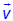: half-arrow above italicized, non-bolded letter
• Magnitude: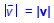• Direction: θ
• Components: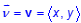• Vector formulas
• If a vector v has components given as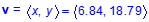, the magnitude and direction can be found using:
• Magnitude: |v| =• Direction: θ = tan-1, when x > 0, or θ = tan-1+ 180°, when x < 0
• If a vector v has a magnitude of |v| and a direction of θ, the components can be found using:
• x = |v|cos θ
• y = |v|sin θ

To Model

SITUATIONS INVOLVING MAGNITUDE AND DIRECTION WITH VECTORS

Including, but not limited to:

• Describing situations with vectors
• Measures of magnitude
• Distance
• Speed (velocity)
• Force
• Measures of direction
• Standard Position Angles

Note(s):

• Geometry used the trigonometric ratios (sine, cosine, and tangent) to determine side lengths and angle measures in right triangles.
• Precalculus uses the trigonometric ratios in order to measure and describe different attributes of vectors.
• Various mathematical process standards will be applied to this student expectation as appropriate.
• TxCCRS:
• II.D. Algebraic Reasoning – Representing relationships
• II.D.2. Convert among multiple representations of equations, inequalities, and relationships.
• III.D. Geometric and Spatial Reasoning – Measurements involving geometry and algebra
• III.D.3. Determine indirect measurements of geometric figures using a variety of methods.
• VII.A. Problem Solving and Reasoning – Mathematical problem solving
• VII.A.3. Determine a solution.
• VIII.B. Communication and Representation – Interpretation of mathematical work
• VIII.B.2. Summarize and interpret mathematical information provided orally, visually, or in written form within the given context.
• IX.A. Connections – Connections among the strands of mathematics
• IX.A.1. Connect and use multiple key concepts of mathematics in situations and problems.
• IX.A.2. Connect mathematics to the study of other disciplines.
• IX.B. Connections – Connections of mathematics to nature, real-world situations, and everyday life
• IX.B.1. Use multiple representations to demonstrate links between mathematical and real-world situations.
• IX.B.2. Understand and use appropriate mathematical models in the natural, physical, and social sciences.
P.4J Represent the addition of vectors and the multiplication of a vector by a scalar geometrically and symbolically.

Represent

THE ADDITION OF VECTORS GEOMETRICALLY AND SYMBOLICALLY

Including, but not limited to:

• Coordinate plane with at least one vector in standard position
• Diagram using horizontal and vertical components
• Symbolically
• If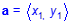and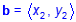, then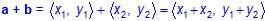• Geometrically
• Head-to-tail (or tip-to-tail) method for adding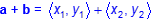• Draw vector a
• Draw vector a + b by connecting the tail of vector a to the head of vector b.
• Parallelogram method for adding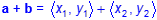• Form a parallelogram using the vectors as adjacent sides.
• Draw vector a + b by drawing the diagonal of the parallelogram.

Represent

THE MULTIPLICATION OF A VECTOR BY A SCALAR GEOMETRICALLY AND SYMBOLICALLY

Including, but not limited to:

• Multiplication of a vector by a scalar
• Coordinate plane with at least one vector in standard position
• Diagram using horizontal and vertical components
• Symbolically
• If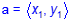, then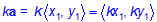• Geometrically
• Head-to-tail (or tip-to-tail) method for finding ka, where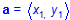• Draw vector a in standard position.
• Copy vector a, starting with the tail at the tip of the original vector a.

Note(s):

• Geometry analyzed lines and segments on the coordinate grid to include distance, midpoint, and slope.
• Precalculus introduces the operations of addition and scalar multiplication of vectors.
• Various mathematical process standards will be applied to this student expectation as appropriate.
• TxCCRS:
• II.D. Algebraic Reasoning – Representing relationships
• II.D.1. Interpret multiple representations of equations, inequalities, and relationships.
• III.A. Geometric and Spatial Reasoning – Figures and their properties
• III.A.2. Form and validate conjectures about one-, two-, and three-dimensional figures and their properties.
• III.C. Geometric and Spatial Reasoning – Connections between geometry and other mathematical content strands
• III.C.1. Make connections between geometry and algebraic equations.
• VII.A. Problem Solving and Reasoning – Mathematical problem solving
• VII.A.3. Determine a solution.
• VIII.B. Communication and Representation – Interpretation of mathematical work
• VIII.B.1. Model and interpret mathematical ideas and concepts using multiple representations.
• VIII.B. Communication and Representation – Interpretation of mathematical work
• VIII.B.2. Summarize and interpret mathematical information provided orally, visually, or in written form within the given context.
• VIII.C. Communication and Representation – Presentation and representation of mathematical work
• VIII.C.1. Communicate mathematical ideas, reasoning, and their implications using symbols, diagrams, models, graphs, and words.
• VIII.C.3. Explain, display, or justify mathematical ideas and arguments using precise mathematical language in written or oral communications.
• IX.A. Connections – Connections among the strands of mathematics
• IX.A.1. Connect and use multiple key concepts of mathematics in situations and problems.
• IX.B.2. Understand and use appropriate mathematical models in the natural, physical, and social sciences.
P.4K Apply vector addition and multiplication of a vector by a scalar in mathematical and real-world problems.

Apply

VECTOR ADDITION AND MULTIPICATION OF A VECTOR BY A SCALAR IN MATHEMATICAL AND REAL-WORLD PROBLEMS

Including, but not limited to:

• Mathematical problem situations
• Combining vectors through addition and scalar multiplication
• Real-world problem situations
• Combining two or more directed distances
• Combining two or more forces

Note(s):

• Geometry used the trigonometric ratios (sine, cosine, and tangent) to determine side lengths and angle measures in right triangles.
• Precalculus uses the trigonometric ratios in order to measure and describe different attributes of vectors.
• Precalculus applies the operations of addition and scalar multiplication of vectors in real-world problems.
• Various mathematical process standards will be applied to this student expectation as appropriate.
• TxCCRS:
• III.D. Geometric and Spatial Reasoning – Measurements involving geometry and algebra
• III.D.3. Determine indirect measurements of geometric figures using a variety of methods.
• VII.A. Problem Solving and Reasoning – Mathematical problem solving
• VII.A.3. Determine a solution.
• VIII.B. Communication and Representation – Interpretation of mathematical work
• VIII.B.2. Summarize and interpret mathematical information provided orally, visually, or in written form within the given context.
• IX.A. Connections – Connections among the strands of mathematics
• IX.A.1. Connect and use multiple key concepts of mathematics in situations and problems.
• IX.A.2. Connect mathematics to the study of other disciplines.
• IX.B. Connections – Connections of mathematics to nature, real-world situations, and everyday life
• IX.B.1. Use multiple representations to demonstrate links between mathematical and real-world situations.
• IX.B.2. Understand and use appropriate mathematical models in the natural, physical, and social sciences.